# Install necessary packages
list_packages <- c("AER", "dynlm", "tidyverse", "fpp", "fpp2",
"quantmod", "urca", "vars", "tseries", "sarima")
new_packages <- list_packages[!(list_packages %in% installed.packages()[,"Package"])]
if(length(new_packages)) install.packages(new_packages)

lapply(list_packages, require, character.only = TRUE)

So far, we have dealt with univariate time series data, what about multiple variables?

# 1 Time Series Regression

The time series models in the previous chapters allow for the inclusion of information from past observations, but not for the inclusion of other variables.

We considered regression models of the form $Z_t=\beta_0 + \beta_1 X_{1,t} + ... \beta_k X_{k,t} + \epsilon_t$

In other words, $$Z_t$$ is a linear combination of $$X_{1,t}$$$$X_{k,t}$$. When $$\epsilon_t$$ is assumed to be an uncorrelated error term (i.e., it is white noise), this is a typical multiple linear regression.

We further allow the errors from a regression to contain autocorrelation. To emphasise this change, we replace $$\epsilon_t$$ with $$\eta_t$$. The error series $$\eta_t$$ is assumed to follow an ARIMA model. For example, if $$\eta_t$$ follows an ARIMA(1,1,1) model, we can write

$Z_t=\beta_0 + \beta_1 X_{1,t} + ... \beta_k X_{k,t} + \eta_t\\ (1-\phi_1 B)(1- B)\eta_t = (1-\theta_1 B) a_t$

Notice that the model has two error terms here — the error from the regression model, which we denote by $$\eta_t$$, and the error from the ARIMA model, which we denote by $$a_t$$. Only the ARIMA model errors are assumed to be white noise.

Note that if $$X_{1,t}$$,…,$$X_{k,t}$$ are excluded from the model, the model becomes an ARIMA model.

## 1.1 Estimation

We cannot use least square estimate for this model because:

• The estimated coefficients are no longer the best estimates, as some information has been ignored in the calculation;
• Any statistical tests associated with the model (e.g., t-tests on the coefficients) will be incorrect. In most cases, the p-values associated with the coefficients will be too small, and so some predictor variables will appear to be important when they are not. This is known as “spurious regression”.
• The AICc values of the fitted models are no longer a good guide as to which is the best model for forecasting.

We use maximum likelihood estimation.

An important consideration when estimating a regression with ARMA errors is that all of the variables in the model must first be stationary. Thus, we first have to check that $$Z_t$$ and all of the predictors appear to be stationary. If we estimate the model when any of these are non-stationary, the estimated coefficients will not be consistent estimates (and therefore may not be meaningful). One exception to this is the case where non-stationary variables are co-integrated. If there exists a linear combination of the non-stationary $$Z_t$$ and the predictors that is stationary, then the estimated coefficients will be consistent. We will explain this later.

It is often desirable to maintain the form of the relationship between $$Z_t$$ and the predictors, and consequently it is common to difference all of the variables if any of them need differencing. The resulting model is then called a “model in differences”, as distinct from a “model in levels”, which is what is obtained when the original data are used without differencing.

If the above regression model with ARIMA(1,1,1) errors is differenced we obtain the model

$\nabla Z_t=\beta_0 + \beta_1 \nabla X_{1,t} + ... \beta_k \nabla X_{k,t} + \nabla \eta_t\\ (1-\phi_1 B) \nabla \eta_t = (1-\theta_1 B) a_t$

R function Arima() will fit a regression model with ARIMA errors if the argument xreg is used. The order argument specifies the order of the ARIMA error model.

The auto.arima() function will also handle regression terms via the xreg argument. The user must specify the predictor variables to include, but auto.arima() will select the best ARIMA model for the errors.

If differencing is required, then all variables are differenced during the estimation process, although the final model will be expressed in terms of the original variables. To include a constant in the differenced model, specify include.drift=TRUE.

Here is an example

We analyze the quarterly changes in personal consumption expenditure and personal disposable income from 1970 to 2016 Q3. We would like to forecast changes in expenditure based on changes in income.

library(fpp2)
data(uschange)
head(uschange)
##         Consumption     Income Production   Savings Unemployment
## 1970 Q1   0.6159862  0.9722610 -2.4527003 4.8103115          0.9
## 1970 Q2   0.4603757  1.1690847 -0.5515251 7.2879923          0.5
## 1970 Q3   0.8767914  1.5532705 -0.3587079 7.2890131          0.5
## 1970 Q4  -0.2742451 -0.2552724 -2.1854549 0.9852296          0.7
## 1971 Q1   1.8973708  1.9871536  1.9097341 3.6577706         -0.1
## 1971 Q2   0.9119929  1.4473342  0.9015358 6.0513418         -0.1
autoplot(uschange[,1:2], facets=TRUE) +
xlab("Year") + ylab("") +
ggtitle("Quarterly changes in US consumption and personal income")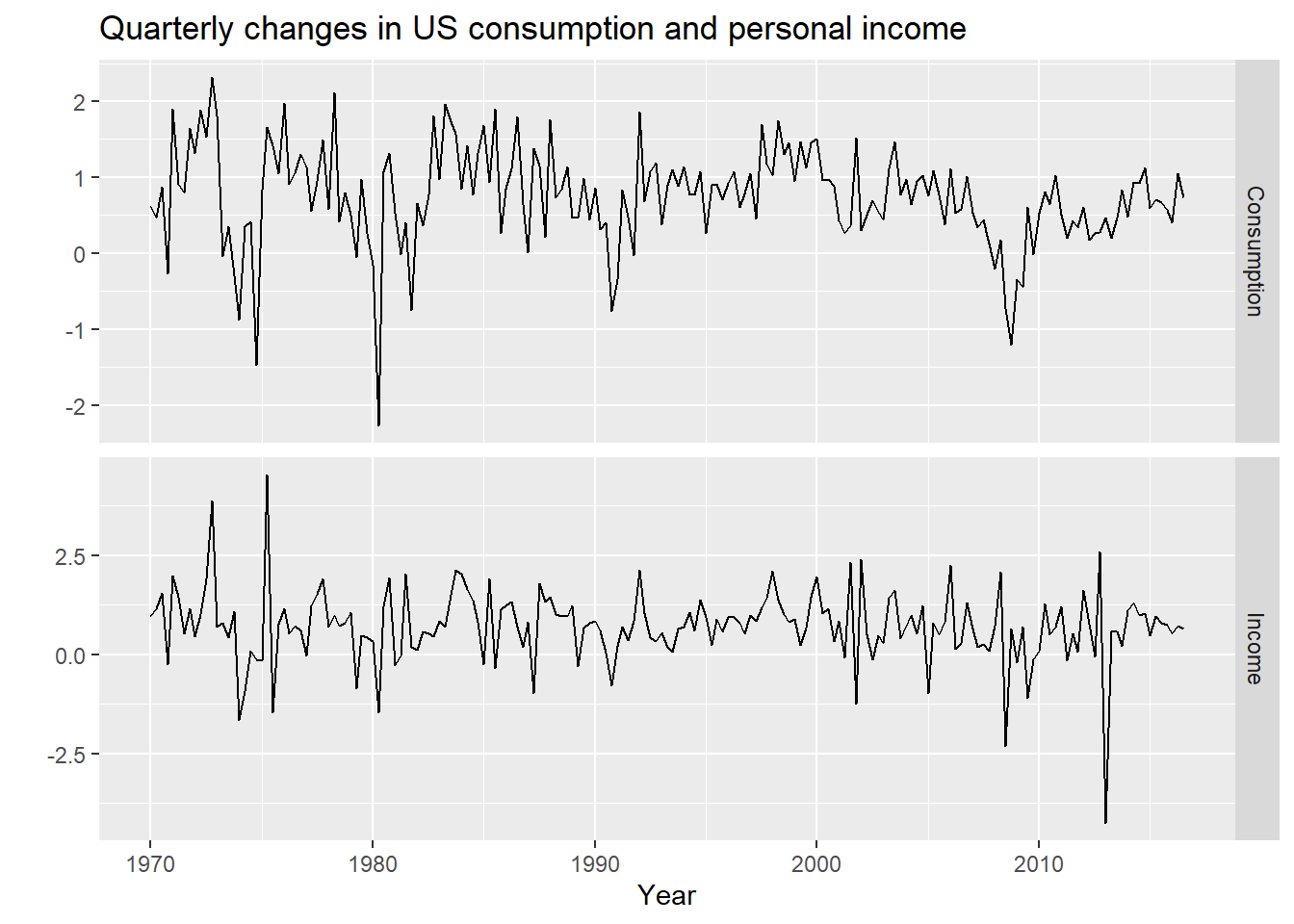The data are clearly already stationary

(fit <- auto.arima(uschange[,"Consumption"], xreg=uschange[,"Income"]))
## Series: uschange[, "Consumption"]
## Regression with ARIMA(1,0,2) errors
##
## Coefficients:
##          ar1      ma1     ma2  intercept    xreg
##       0.6922  -0.5758  0.1984     0.5990  0.2028
## s.e.  0.1159   0.1301  0.0756     0.0884  0.0461
##
## sigma^2 estimated as 0.3219:  log likelihood=-156.95
## AIC=325.91   AICc=326.37   BIC=345.29
(fit <- Arima(uschange[,"Consumption"], order=c(1,0,2), xreg=uschange[,"Income"]))
## Series: uschange[, "Consumption"]
## Regression with ARIMA(1,0,2) errors
##
## Coefficients:
##          ar1      ma1     ma2  intercept    xreg
##       0.6922  -0.5758  0.1984     0.5990  0.2028
## s.e.  0.1159   0.1301  0.0756     0.0884  0.0461
##
## sigma^2 estimated as 0.3219:  log likelihood=-156.95
## AIC=325.91   AICc=326.37   BIC=345.29

The fitted model is

$Z_t = 0.599 + 0.203 X_t + \eta_t,\\ \eta_t = 0.692 \eta_{t-1} + a_t + 0.576 a_{t−1} - 0.198 a_{t−2}$

where $$a_t$$ is white noise.

We can recover estimates of both the $$\eta_t$$ and $$a_t$$ series using the residuals(). function.

cbind("Regression Errors" = residuals(fit, type="regression"),
"ARIMA errors" = residuals(fit, type="innovation")) %>%
autoplot(facets=TRUE)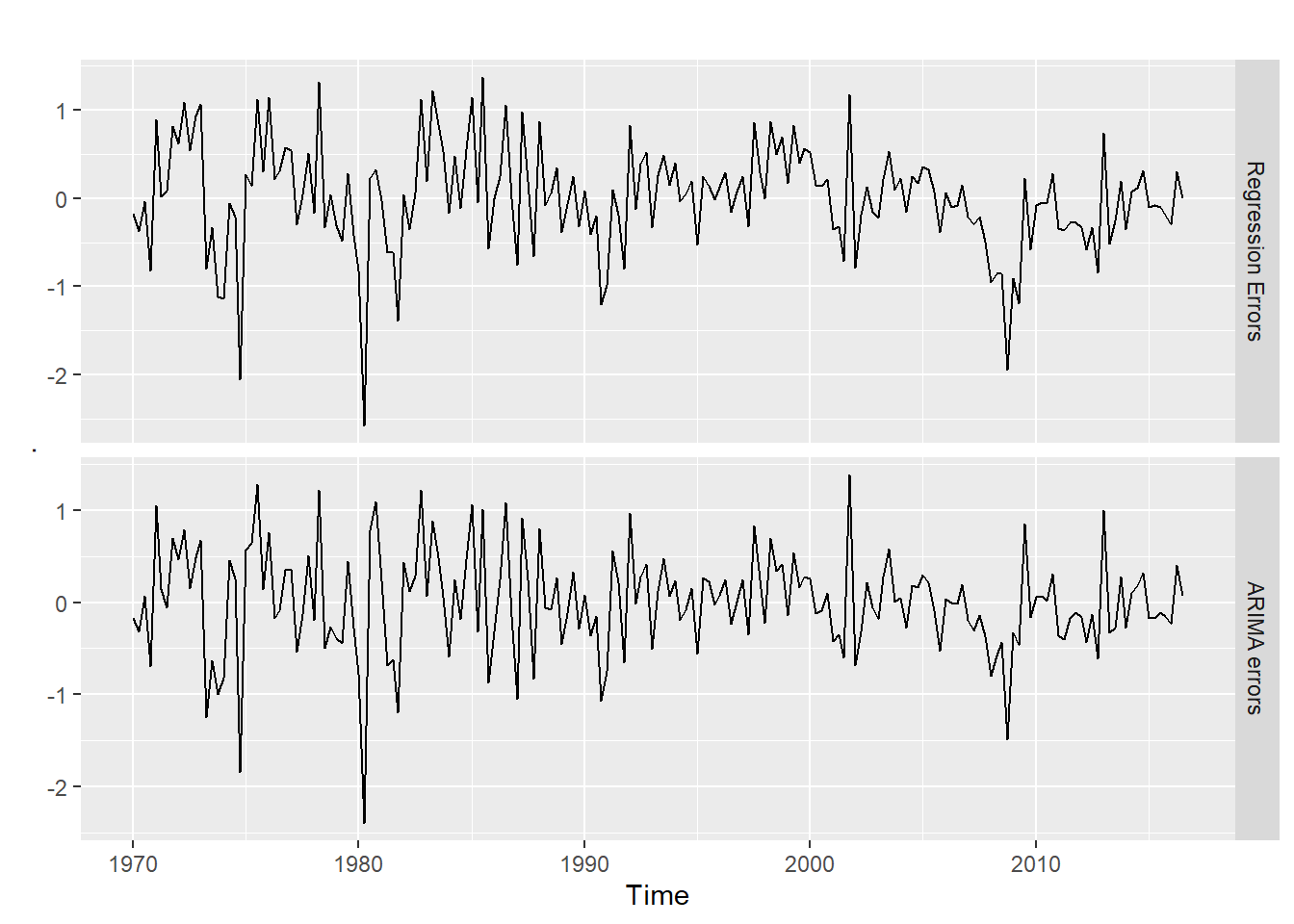It is the ARIMA errors that should resemble a white noise series.

checkresiduals(fit)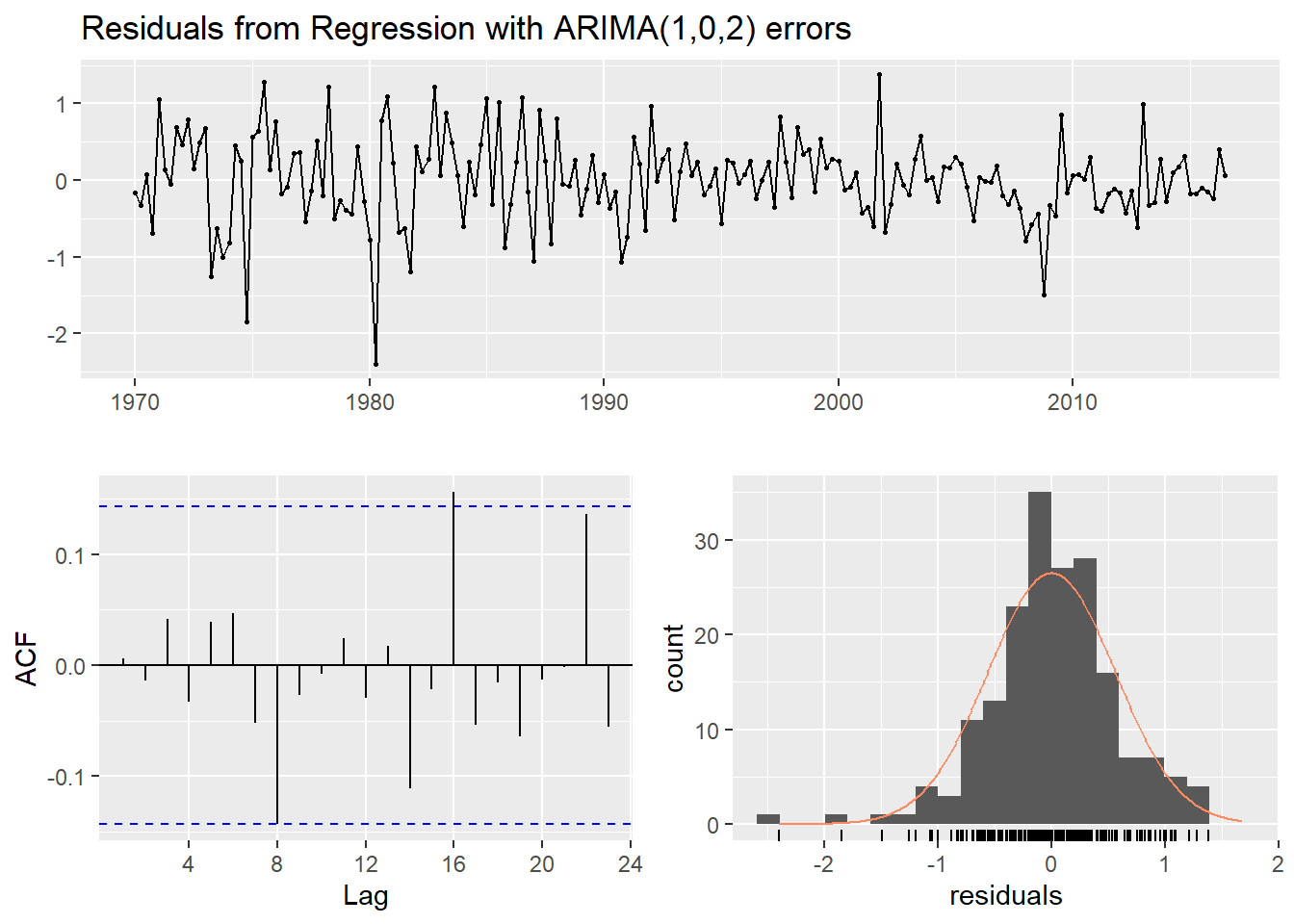##
##  Ljung-Box test
##
## data:  Residuals from Regression with ARIMA(1,0,2) errors
## Q* = 5.8916, df = 3, p-value = 0.117
##
## Model df: 5.   Total lags used: 8
uschange %>%
as.data.frame() %>%
ggplot(aes(x=Income, y=Consumption)) +
ylab("Consumption (quarterly % change)") +
xlab("Income (quarterly % change)") +
geom_point() +
geom_smooth(method="lm", se=FALSE)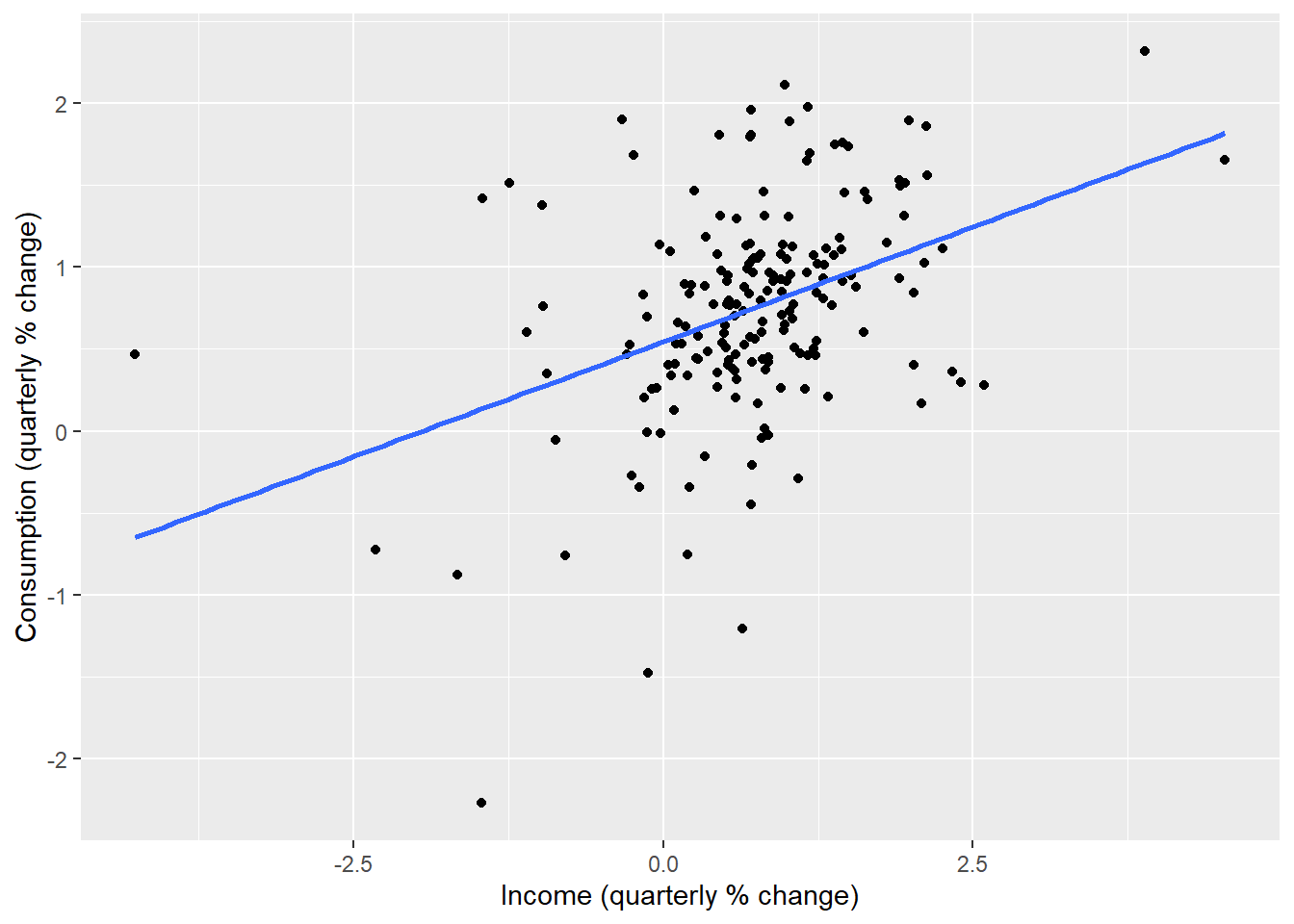## 1.2 Forecast

To forecast using a regression model with ARIMA errors, we need to forecast the regression part of the model and the ARIMA part of the model, and combine the results.

When the predictors are themselves unknown, we must either model them separately, or use assumed future values for each predictor.

We will calculate forecasts for the next eight quarters assuming that the future percentage changes in personal disposable income will be equal to the mean percentage change from the last forty years.

fcast <- forecast(fit, xreg=rep(mean(uschange[,2]),20))
autoplot(fcast) + xlab("Year") +
ylab("Percentage change")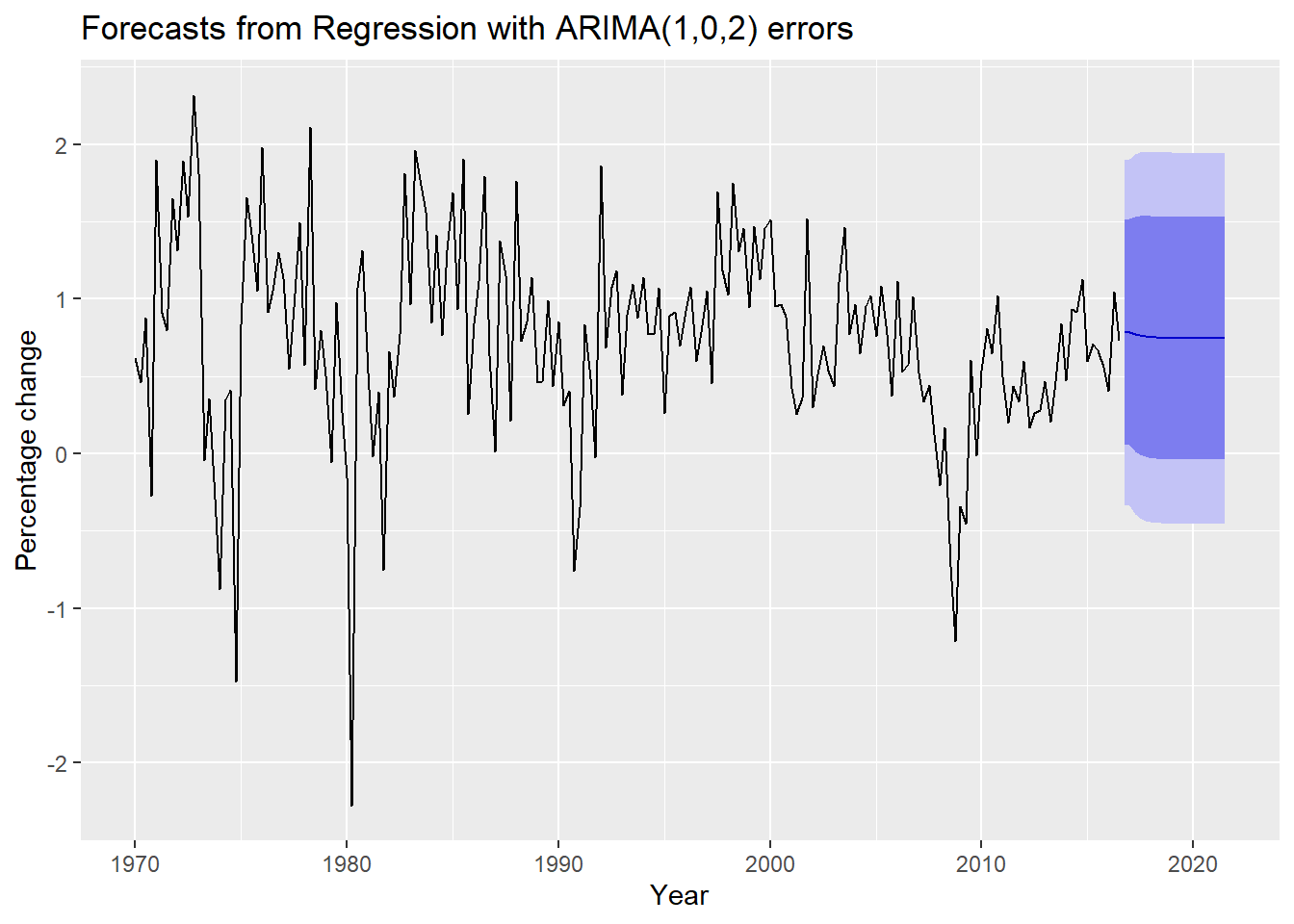The prediction intervals for this model are narrower than the ARIMA model for $$Z_t$$ because we are now able to explain some of the variation in the data using the income predictor.

fit2 <- Arima(uschange[,"Consumption"], order=c(1,0,2))
fcast2 <- forecast(fit2,h=20)
autoplot(fcast2) + xlab("Year") +
ylab("Percentage change")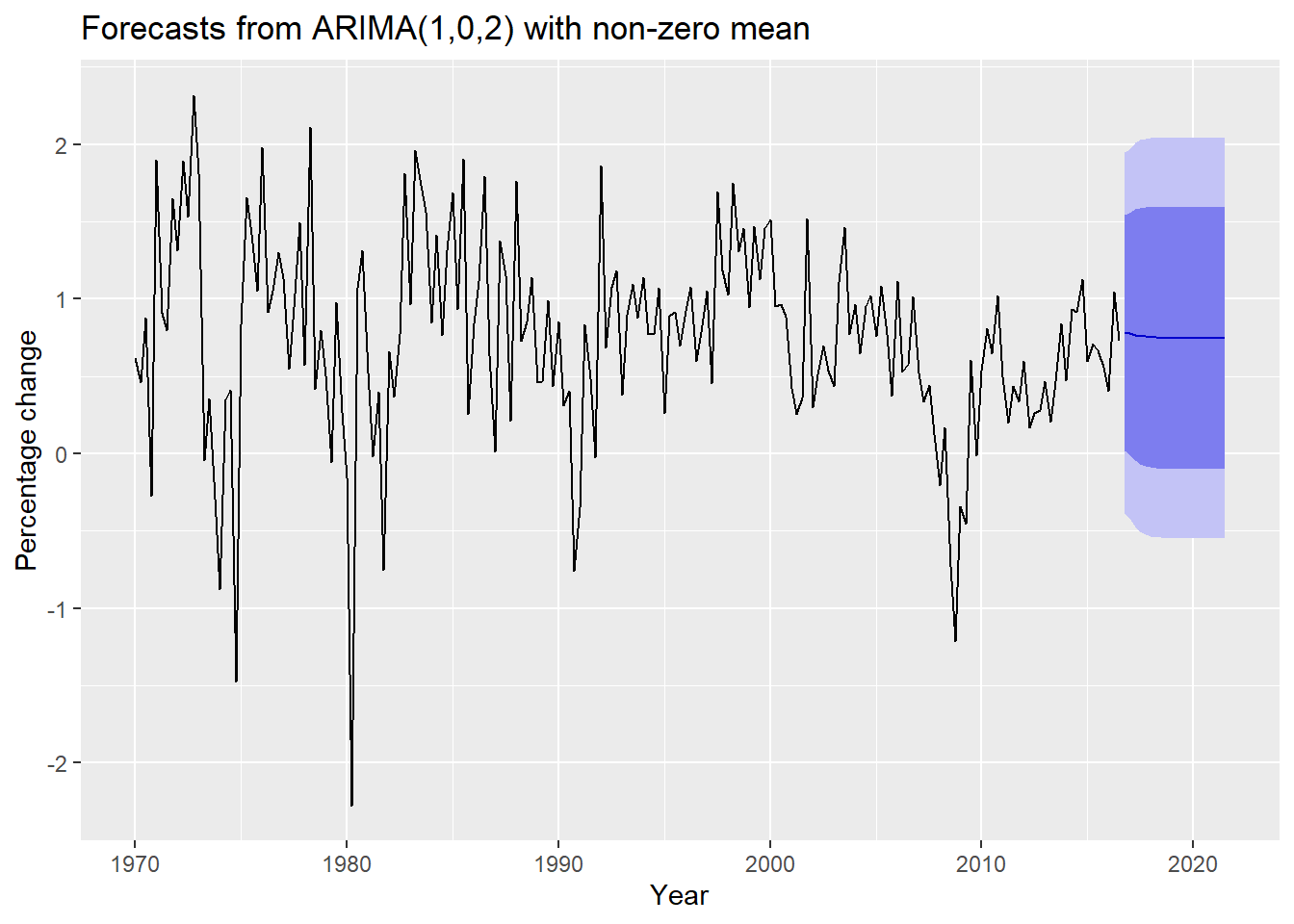It is important to realise that the prediction intervals from regression models (with or without ARIMA errors) do not take into account the uncertainty in the forecasts of the predictors. So they should be interpreted as being conditional on the assumed (or estimated) future values of the predictor variables.

## 1.3Gasoline price

Suppose we are interested in the level of gas prices (Data) as a function of various explanatory variables.

We observe Gas Prices $$Y_t$$ over $$n$$ time periods, $$t = 1, 2, \dots, n$$.

1. we can forecast $$Y$$ purely against time and itself;

2. Or, we can apply a VAR by using possible variables: Personal Disposable Income Consumer Price Index Unemployment Housing Starts Price of crude oil

# my_data1 <- Gas_prices[,-c(1:3,10:29)]
# Import the data ---------------------------------------------------------
head(GasPrices)
## # A tibble: 6 x 29
##   Date   Year Month Unleaded Crude_price SP500   PDI   CPI RR_sales Unemp
##   <chr> <dbl> <dbl>    <dbl>       <dbl> <dbl> <dbl> <dbl>    <dbl> <dbl>
## 1 15-J~  1996     1     109.        18.9  636. 5660   154.   134926   5.6
## 2 15-F~  1996     2     109.        19.1  640. 5713.  155.   136781   5.5
## 3 15-M~  1996     3     114.        21.3  646. 5749.  156.   137527   5.5
## 4 15-A~  1996     4     123.        23.5  654. 5733.  156.   137504   5.6
## 5 15-M~  1996     5     128.        21.2  669. 5816   157.   138302   5.6
## 6 15-J~  1996     6     126.        20.4  671. 5851.  157.   137881   5.3
## # ... with 19 more variables: Housing <dbl>, Demand <dbl>, Production <dbl>,
## #   Imports <dbl>, Stocks <dbl>, Time <dbl>, L1_Unleaded <dbl>,
## #   L1_Crude_price <dbl>, L1_SP500 <dbl>, L1_PDI <dbl>, L1_CPI <dbl>,
## #   L1_RR_sales <dbl>, L1_Unemp <dbl>, L1_Housing <dbl>, L1_Demand <dbl>,
## #   L1_Production <dbl>, L1_Imports <dbl>, L1_Stocks <dbl>, Recession <dbl>
if (!require("forecast")){install.packages("forecast")}
Unleaded <- ts(GasPrices$Unleaded, start=1996, frequency = 12, end=c(2015, 12)) # Transformation---------------------------------------------------------- log_Unleaded <- log(Unleaded) # auto.arima fit.auto <- auto.arima(log_Unleaded) print(fit.auto) ## Series: log_Unleaded ## ARIMA(0,1,1) ## ## Coefficients: ## ma1 ## 0.5588 ## s.e. 0.0488 ## ## sigma^2 estimated as 0.003014: log likelihood=354.82 ## AIC=-705.64 AICc=-705.59 BIC=-698.69 checkresiduals(fit.auto)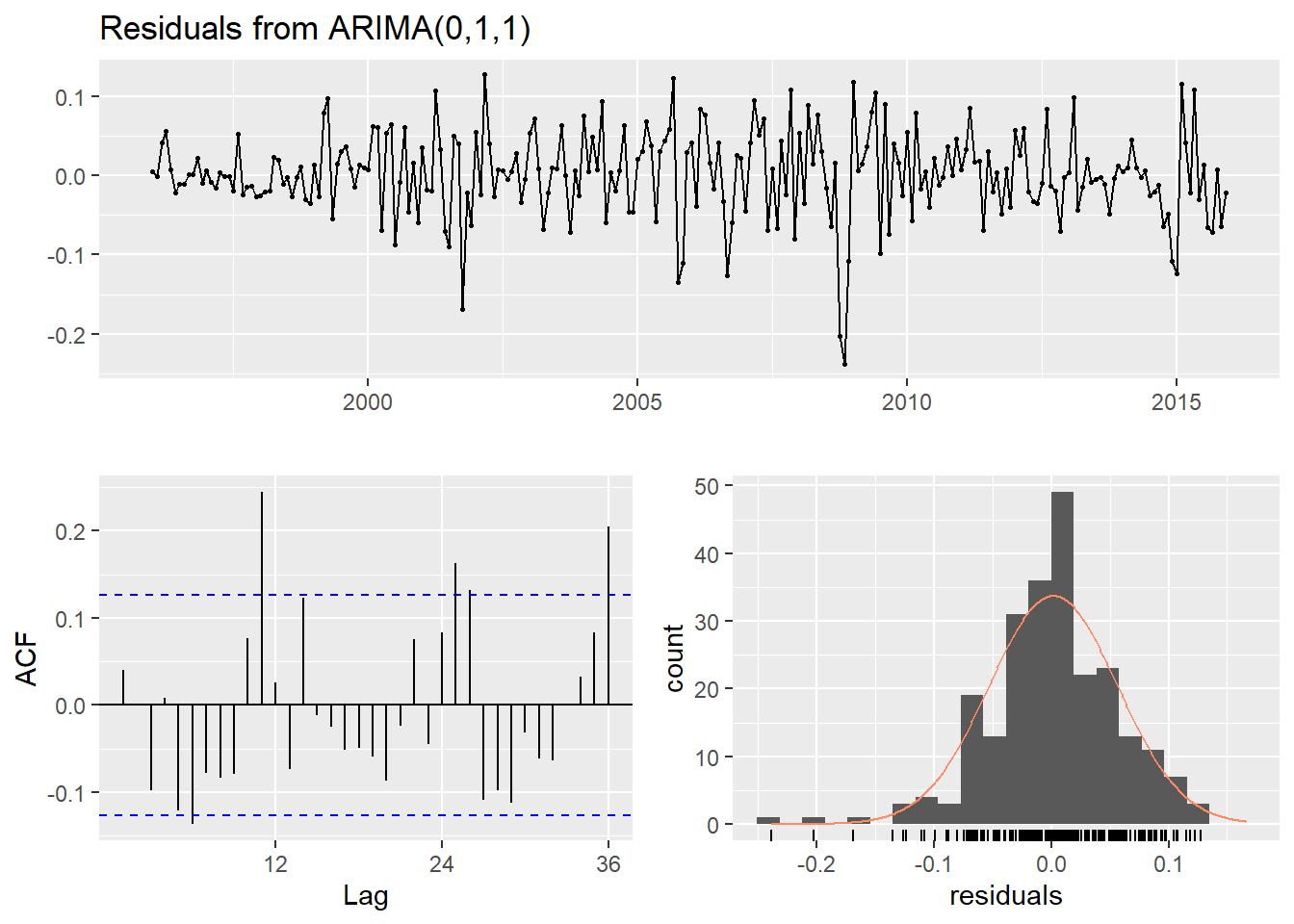## ## Ljung-Box test ## ## data: Residuals from ARIMA(0,1,1) ## Q* = 46.458, df = 23, p-value = 0.002622 ## ## Model df: 1. Total lags used: 24 mod1 <- lm(Unleaded~Crude_price + CPI + Demand, data = GasPrices) summary(mod1) ## ## Call: ## lm(formula = Unleaded ~ Crude_price + CPI + Demand, data = GasPrices) ## ## Residuals: ## Min 1Q Median 3Q Max ## -31.549 -10.814 -1.954 7.357 65.137 ## ## Coefficients: ## Estimate Std. Error t value Pr(>|t|) ## (Intercept) -48.26392 19.52878 -2.471 0.0142 * ## Crude_price 2.30659 0.06870 33.575 < 2e-16 *** ## CPI 0.76253 0.09357 8.149 2.14e-14 *** ## Demand -0.14675 0.14335 -1.024 0.3070 ## --- ## Signif. codes: 0 '***' 0.001 '**' 0.01 '*' 0.05 '.' 0.1 ' ' 1 ## ## Residual standard error: 16.58 on 236 degrees of freedom ## Multiple R-squared: 0.9681, Adjusted R-squared: 0.9677 ## F-statistic: 2386 on 3 and 236 DF, p-value: < 2.2e-16 fit <- auto.arima(GasPrices$Unleaded, xreg=as.matrix(GasPrices[,c("Crude_price", "CPI", "Demand")]))
summary(fit)
## Series: GasPrices\$Unleaded
## Regression with ARIMA(1,0,2) errors
##
## Coefficients:
##          ar1     ma1     ma2  intercept  Crude_price     CPI  Demand
##       0.7134  0.4971  0.1281  -207.2742       1.6327  1.6563  0.3211
## s.e.  0.0955  0.0997  0.0950    57.5287       0.1951  0.3035  0.2907
##
## sigma^2 estimated as 92.26:  log likelihood=-880.77
## AIC=1777.55   AICc=1778.17   BIC=1805.39
##
## Training set error measures:
##                       ME     RMSE      MAE        MPE     MAPE      MASE
## Training set -0.06211942 9.463917 6.632496 -0.1367517 3.007046 0.6452159
##                     ACF1
## Training set -0.00322775
checkresiduals(fit)